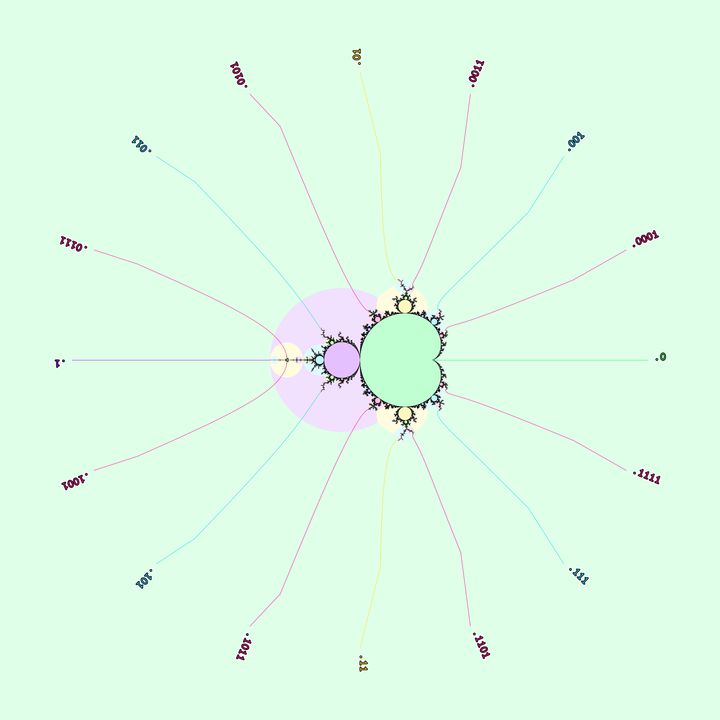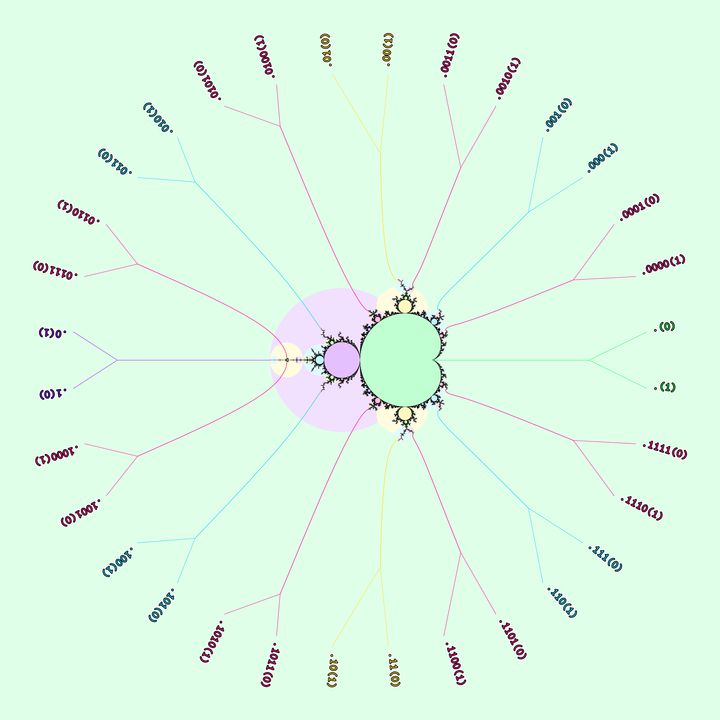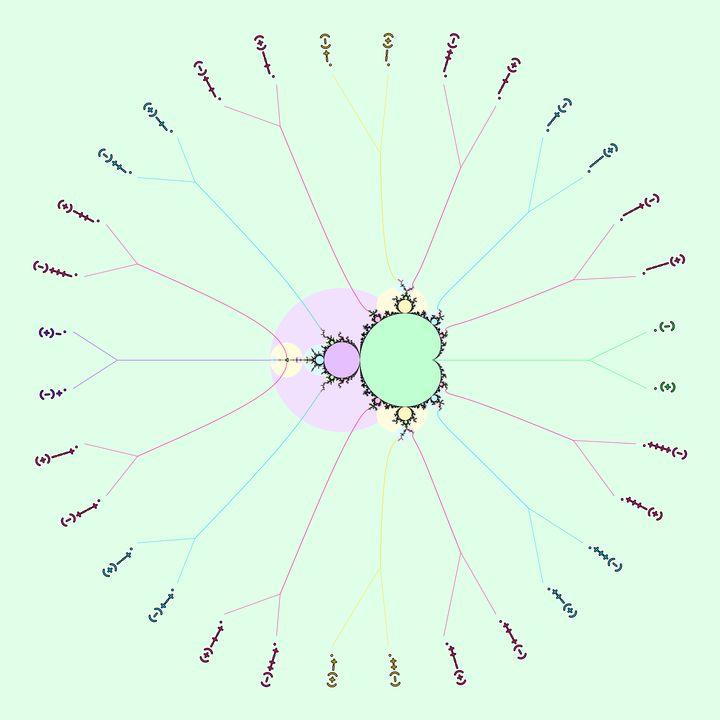# mathr / blog / #

## Islands in the hairs

In a previous post I explored patterns of periods in the Mandelbrot set and promised a future post elaborating on external angles.

Here are some external rays landing on tips relative to the period 1 continent of the Mandelbrot set, with their angles labeled in turns using a finite binary expansion:Actually, a finite binary expansion is really an infinite binary expansion, ending in an infinite string of 0s. But there's another infinite binary expansion for each number ending in infinite 0s, that ends in an infinite string of 1s: .xyz1000... = .xyz0111.... Writing down an infinite string is boring, so I write the repeated part in (parentheses).Now we can get more abstract, replace "0" with "-" and "1" with "+":The tuning algorithm for external angles works like this: start with a pair of periodic external angles (.(-),.(+)) whose rays land at the root of the same hyperbolic component H, and another external angle E. Now replace every 0 in E with -, and every 1 in E with +, to form the external angle F. Then F's ray lands near H in the same way that E's ray lands near the period 1 continent.

For example, the root of the period 3 island has external angles (.(011),.(100)), and the 1/2 bulb of the period 1 cardioid has external angles (.(01), (.10)). Applying the tuning algorithm, the 1/2 bulb of the period 3 cardioid has external angles (.(011 100),.(100 011)).

Now the two binary representations come in to play: for each tip, applying tuning gives two unequal angles with rays that land on the same point. Between the two angles there must be more of the Mandelbrot set, so it must be quite a hairy beast.

Some examples of hairiness:

There are islands in the hairs (because there are islands everywhere, though they get very small very quickly), and in the previous post I noted that around a period P island the periods in each successive layer of hairs seems to increase by P each time. Now I make that more precise.

Suppose we have an island I0 with angles (.(-),.(+)). Then the tip of its antenna has angles (.-(+),.+(-)). There will be more islands in the wake of the antenna. Suppose I1 is the lowest period island visible in the wake (visible means it isn't separated from the viewpoint by a ray-pair of lower period). I1 is in fact defined uniquely:

Assume there are two lowest-period visible islands with p = period(I1a) = period(I1b). So there are 4 rays of period p landing on I1a and I1b in the wake. Neighbouring rays of period p are separated by 1/(2^p - 1) so the total width of the wake must be more than 3/(2^p - 1). But: 3/(2^p - 1) is more than 1/(2^(p-1) - 1) when p > 2 so there is room for a period (p-1) island in the wake, which contradicts the assumption.

So, the width W of the wake satisfies: 1/(2^p-1) < W < 1/(2^(p-1)-1), and p > 2. The lower bound is .0...01(0...01) and the upper bound is .0...1(00...1), where the group of 3 digits surrounds the p'th digit. Because of the strict inequalities, the position of the first 1 in the digits of W must be at the p'th digit - if it was any different it would be outside the bounds.

Brief recap: given the roots of an island, find the wake of the tip, then the lowest period island has period of the first index of 1 in the digits of the width of the wake. Call this period p, and the angles of the wake (W-,W+) then the island in the hair has angles (ceil[(2^p-1)(W-)]/(2^p-1), floor[(2^p-1)(W+)]/ (2^p-1)) (Proof left as an exercise because I don't feel like typing it up: I used the fact that (2^p-1)(W-) is not an integer).

So far this is just the hair of the antenna, but there are hairs all over. Because of tuning, the width of the wakes of each successive layer of hairs on a period P island is 1/2^P times the previous layer. The lowest period in the antenna hair is p, and call the period in the next layer of hairs p'. Now algebraic manipulation shows that if the wake W is wide enough for p then the wake W' in the next level is wide enough for an island of period p+P, and a proof by contradiction shows that W' is not wide enough for an island of period p+P-1. Combining with the earlier uniqueness, the lowest period islands in the next level of hairs must have period p' = p + P.